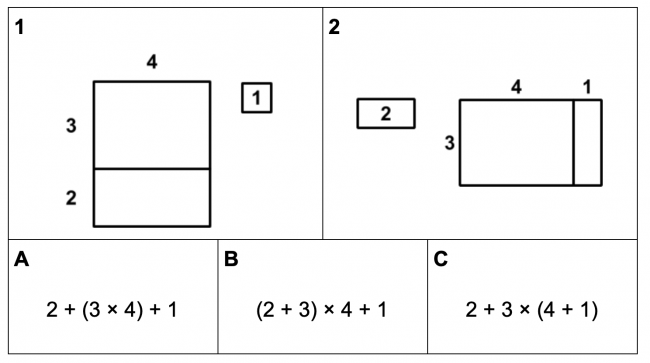# Order of Operations

Preview:This task helps students visualize the relationship between finding area and order of operations.

Connecting Representations
Big Idea:
Grade 6: Computational fluency and flexibility with numbers extend to operations with whole numbers and decimals.
Curricular Competency:
Reasoning and analyzing: Use reasoning to explore and make connections
Reasoning and analyzing: Develop mental math strategies and abilities to make sense of quantities
Understanding and solving: Develop, demonstrate, and apply mathematical understanding through play, inquiry, and problem solving
Understanding and solving: Visualize to explore mathematical concepts
Communicating and representing: Communicate mathematical thinking in many ways